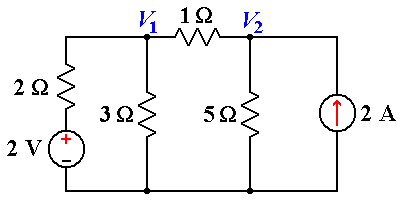# DC circuits analysis using mesh & nodal method

Jan 17 • General • 5880 Views • 3 Comments on DC circuits analysis using mesh & nodal method

The Mesh Method:

The Mesh method uses the Mesh currents as the circuit variables. The procedure for Obtaining the solution is similar to that followed in the Node method and the various Steps are given below.

1. Clearly label all circuit parameters and distinguish the unknown parameters from the known.

2. Identify all meshes of the circuit.

3. Assign mesh currents and label polarities.

4. Apply KVL at each mesh and express the voltages in terms of the mesh currents.

5. Solve the resulting simultaneous equations for the mesh currents.

6. Now that the mesh currents are known, the voltages may be obtained from Ohm’s law.

A mesh is defined as a loop which does not contain any other loops.

Mesh Analysis CircuitOne simple method of reducing the amount of math’s involved is to analyse the circuit using Kirchhoff’s Current Law equations to determine the currents, I1 and I2 flowing in the two resistors. Then there is no need to calculate the current I3 as its just the sum of I1 and I2. So Kirchhoff’s second voltage law simply becomes:

• Equation No 1 :    10 =  50I1 + 40I2
• Equation No 2 :    20 =  40I1 + 60I2

Therefore, one line of math’s calculation has been saved.

Mesh Current Analysis

• An easier method of solving the above circuit is by using Mesh Current Analysis or Loop Analysis which is also sometimes called Maxwell´s Circulating Currents method.

Instead of labeling the branch currents we need to label each “closed loop” with a circulating current. As a general rule of thumb, only label inside loops in a clockwise direction with circulating currents as the aim is to cover all the elements of the circuit at least once. Any required branch current may be found from the appropriate loop or mesh currents as before using Kirchhoff’s method.

EXAMPLE–For example:    i1 = I1, i2 = -I2 and I3 = I1 – I2

We now write Kirchhoff’s voltage law equation in the same way as before to solve them but the advantage of this method is that it ensures that the information obtained from the circuit equations is the minimum required to solve the circuit as the information is more general and can easily be put into a matrix form.

For example, consider the circuit from the previous section.

These equations can be solved quite quickly by using a single mesh impedance matrix Z. Each element ON the principal diagonal will be “positive” and is the total impedance of each mesh. Where as, each element OFF the principal diagonal will either be “zero” or “negative” and represents the circuit element connecting all the appropriate meshes. This then gives us a matrix of:

Where:  [V]= [R] [ I ]. Then it can be solved by matrix method.

• [V]   Gives the total battery voltage for loop 1 and then loop 2.
• [I]     states the names of the loop currents which we are trying to find.
• [R]   Is called the resistance matrix.

And this gives I1 as -0.143 Amps and I2 as -0.429 Amps

As:    I3 = I1 – I2.The current I3 is therefore given as:    -0.143 – (-0.429) = 0.286 Amps.

The Node Method.

A voltage is always defined as the potential difference between two points. When we talk about the voltage at a certain point of a circuit we imply that the measurement is performed between that point and some other point in the circuit. In most cases that other point is referred to as ground.

•   The node method or the node voltage method, is a very powerful approach for circuit analysis and it is based on the application of KCL, KVL and Ohm’s law. The procedure for analyzing a circuit with the node method is based on the following steps.

1. Clearly label all circuit parameters and distinguish the unknown parameters from the known.

2. Identify all nodes of the circuit.

3. Select a node as the reference node also called the ground and assign to it a potential of 0 Volts. All other voltages in the circuit are measured with respect to the reference node.

4. Label the voltages at all other nodes.

5. Assign and label polarities.

6. Apply KCL at each node and express the branch currents in terms of the node voltages.

7. Solve the resulting simultaneous equations for the node voltages.6.071, Spring 2006. Chaniotakis and Cory 18. Now that the node voltages are known, the branch currents may be obtained from Ohm’s law.

EXAMPLE —   What is the voltage across the current source? Via nodal analysis:

Defining the nodal voltages in the conventional way (with the reference node at the bottom grounded to 0 V) leads to:KCL at node 1:

(V1-2)/2 + V1/3 + (V1-V2) = 0

KCL at node 2:

(V2-V1) + V2/5 -2 = 0

Multiplying eqn. 1 through by 6, eqn. 2 through by 5, and consolidating terms leads to the following two equations to solve:

11 V1 – 6 V2 = 6

-5 V1 + 6 V2 = 10